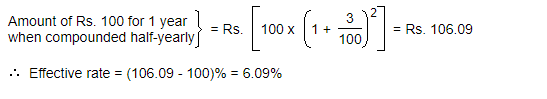# Quantitative Aptitude Quiz for SSC: 26 March 2021

Updated Fri, 26 Mar 2021 07:51 PM IST

1. A bank offers 5% compound interest calculated on half-yearly basis. A customer deposits Rs. 1600 each on 1st January and 1st July of a year. At the end of the year, the amount he would have gained by way of interest is:

a) Rs.Source: safalta.com

110
b) Rs. 121
c) Rs. 132
d) Rs.

### Free Demo Classes

143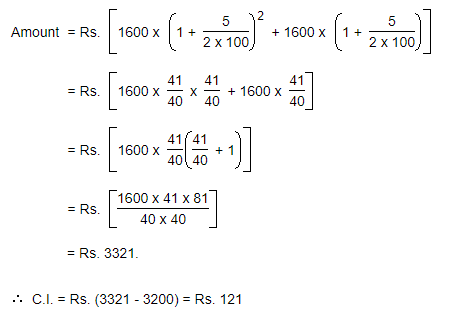2. The difference between simple and compound interests compounded annually on a certain sum of money for 2 years at 4% per annum is Re. 1. The sum (in Rs.) is:

a) 600
b) 625
c) 650
d) 675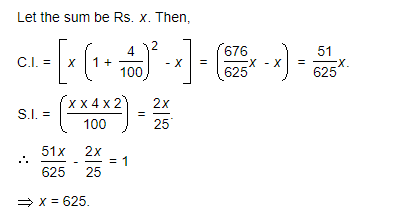3. There is 60% increase in an amount in 6 years at simple interest. What will be the compound interest of Rs. 12,000 after 3 years at the same rate?

a) 3972
b) 3978
c) 3996
d) 3948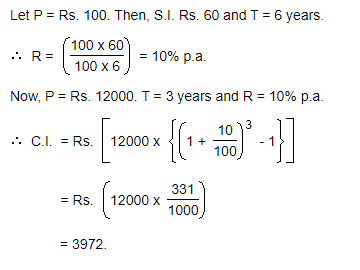4. What is the difference between the compound interests on Rs. 5000 for 3/2 years at 4% per annum compounded yearly and half-yearly?

a) Rs, 4.24
b) Rs. 5.72
c) Rs. 2.04
d) Rs. 3.24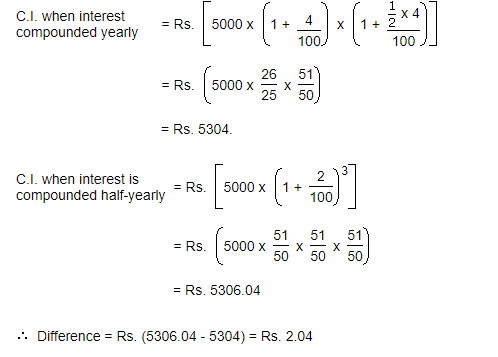5. The compound interest on Rs. 30,000 at 7% per annum is Rs. 4347. The period (in years) is:

a) 1 year
b) 3/2 year
c) 2 year
d) 5/2 year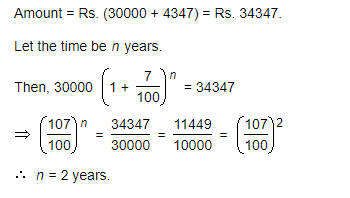6. What will be the compound interest on a sum of Rs. 25,000 after 3 years at the rate of 12 p.a.?

a) Rs. 10123.20
b) Rs. 11023.20
c) Rs. 10213.20
d) Rs. 12103.20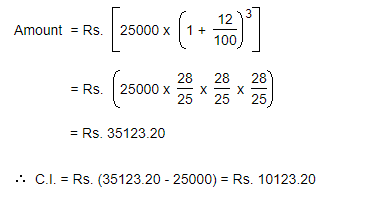7. At what rate of compound interest per annum will a sum of Rs. 1200 become Rs. 1348.32 in 2 years?

a) 4%
b) 6%
c) 8%
d) 10%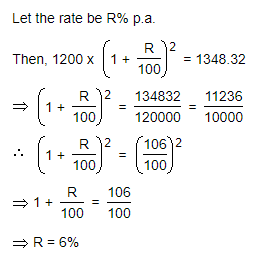8. The least number of complete years in which a sum of money put out at 20% compound interest will be more than doubled is:

a) 2
b) 3
c) 4
d) 5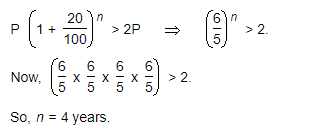9. Albert invested an amount of Rs. 8000 in a fixed deposit scheme for 2 years at compound interest rate 5 p.c.p.a. How much amount will Albert get on maturity of the fixed deposit?

a) 8820
b) 8640
c) 8780
d) 8960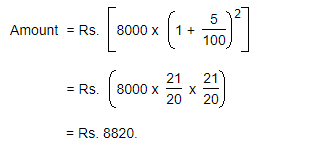10. The effective annual rate of interest corresponding to a nominal rate of 6% per annum payable half-yearly is:

a) 6.16%
b) 6.09%
c) 6.26%
d) 6.49%